Calculus of Variations: Numerical Solution of th Euler Equation for the Brachiostone

This is the form of the total time's integrand as derived above for v(s) = cos(α)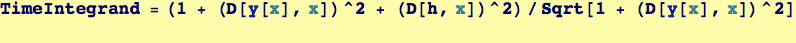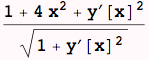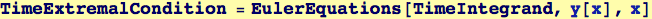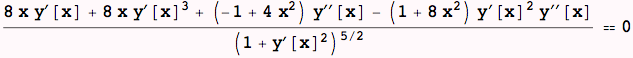This ODE doesn't have a closed-form solution; so we find a numerical approximation to the solution to the Euler equation, extract it and then plot it.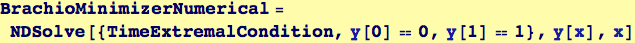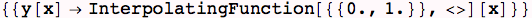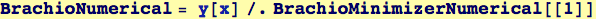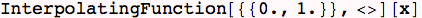Created by Wolfram Mathematica 6.0  (26 November 2007)